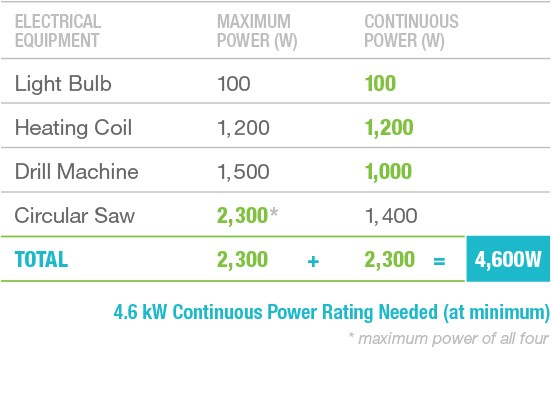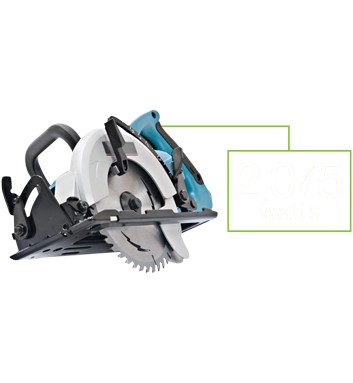# Power on the Go.

Just scroll down the page to get all the info you need
on portable generators.All you need to do is some good ol’ grade school math. First, list out all the items you want to power.

Find the item with the most Maximum Power (aka Starting Watts). Then add that number (2,300 W) to the Continuous Power (Running Watts) from all the other units (100 + 1,200 + 1,000). For this example, the total is 4,600 W. So you’ll need a generator with a Continuous Power rating of at least a 4.6 kW (4,600 W).

Note: only equipment with electric motors requires more power to start than run.

# How is aportable generator rated?

All portable generators have two power ratings: Continuous Power (aka Running Watts) and Maximum Power (aka Starting Watts).

Running Watts are what the generator can produce continuously while it’s running.

Starting Watts are what it can produce for a short time to help start motorized electrical tools like drill machines, circular saws, nail guns, etc.

# What’s a Watt?

CLOSE# What’s a Watt?

A ‘Watt’ (denoted with a capital ‘W’ after James Watt) is a unit of electrical energy. You can find Wattage estimates on the labels of your tools.

If you can’t find the wattage amount, don’t worry. All you need to know is the volts and amps of your product. To calculate the wattage, simply multiply volts X amps. So a circular saw that operates on 110 volts and consumes 20 amps needs 2,200 Watts to run and requires a generator with a Continuous Power rating of at least 2.2kW (2,200 Watts).